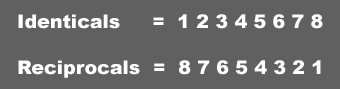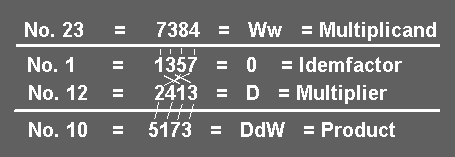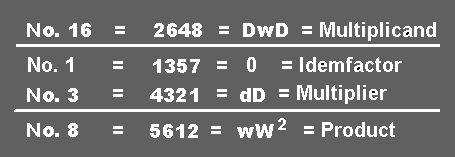Begin by comparing each digit of the Multiplier with those of the Idemfactor. For every digit in the one there will be an identical numeral or a corresponding reciprocal in the other. When there is not an identical parity match between numerals, the reciprocal is used, which is the cubic diagonal opposite of the numeral in question. For example, the reciprocal of 1 is 8, of 2 is 7, of 3 is 6 and of 4 is 5 and vice versa for the remaining numerals. See the full matching example in the chart below:In working the algorithm, the Product is set down directly below each numeral in the Multiplier. The Product numeral is the same digit as that which is directly above the Idemfactor identical or reciprocal numeral matched with each digit of the Multiplier. For instance, in the previous example of the Algorithm, we look at the Multiplier 2413 and begin by matching the 1 digit with its respective 1 in the Idemfactor. Directly above the Idemfactor 1 we see the 7 from our Multiplicand, which is brought down directly underneath the 1 in the Multiplier as our Product. likewise, the 3 in the Multiplier matched with the 3 in the Idemfactor results in a Product of 3 brought down from the Multiplicand.Now, there are no more identical matches between the multiplier and Idemfactor digits, so the 2 in the Multiplier is matched with its reciprocal 7 in the Idemfactor, consequently, when we bring down the Product digit from the Multiplicand above the 7, we use the reciprocal of that digit (4), which is a 5. Lastly, we match the 4 of the Multiplier with its reciprocal 5 in the Idemfactor and bring down its reciprocal digit (1) from the Multiplicand 8 and place the 1 as the Product beneath the 4 of the Multiplier. This process is duplicated in the example above for clarity.

Another example of the Tropic Algorithm is shown below using the #16 Exact Face as the Multiplicand and the #3 Exact Face as its Multiplier. In the Multiplier, we see an identical 3 above it and bring down the 6. The 1 has an identical also and thus bring down the 2 of the Multiplicand into the Product. The 4 finds its reciprocal in the 5 and brings down a 5 into the Product. And lastly, the 2 finds its reciprocal in 7 and brings down the reciprocal digit from the Multiplicand into the Product.In this manner one can easily draw a proportional algorithm from any given Exact Face of one tablock to any other. Let's take an example next which involves reflection from one tablock to another in addition to turning.

NEXT